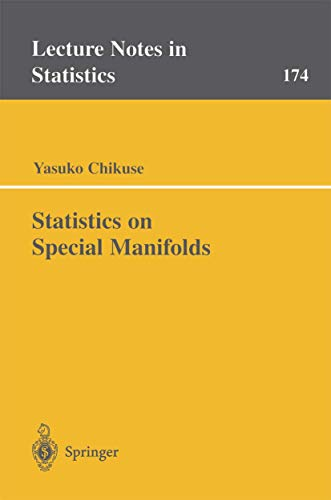Statistics on Special Manifolds (Lecture Notes in Statistics)

Yasuko Chikuse

0 durchschnittliche Bewertung
( 0 Bewertungen bei Goodreads )Alle Exemplare der Ausgabe mit dieser ISBN anzeigen:

Book by Chikuse Yasuko

Die Inhaltsangabe kann sich auf eine andere Ausgabe dieses Titels beziehen.

Reseþa del editor:

Covering statistical analysis on the two special manifolds, the Stiefel manifold and the Grassmann manifold, this book is designed as a reference for both theoretical and applied statisticians. It will also be used as a textbook for a graduate course in multivariate analysis. It is assumed that the reader is familiar with the usual theory of univariate statistics and a thorough background in mathematics, in particular, knowledge of multivariate calculation techniques.

This book is concerned with statistical analysis on the two special manifolds, the Stiefel manifold and the Grassmann manifold, treated as statistical sample spaces consisting of matrices. The former is represented by the set of m x k matrices whose columns are mutually orthogonal k-variate vectors of unit length, and the latter by the set of m x m orthogonal projection matrices idempotent of rank k. The observations for the special case k=1 are regarded as directed vectors on a unit hypersphere and as axes or lines undirected, respectively. Statistical analysis on these manifolds is required, especially for low dimensions in practical applications, in the earth (or geological) sciences, astronomy, medicine, biology, meteorology, animal behavior and many other fields. The Grassmann manifold is a rather new subject treated as a statistical sample space, and the development of statistical analysis on the manifold must make some contributions to the related sciences. The reader may already know the usual theory of multivariate analysis on the real Euclidean space and intend to deeper or broaden the research area to statistics on special manifolds, which is not treated in general textbooks of multivariate analysis.

The author rather concentrates on the topics to which a considerable amount of personal effort has been devoted. Starting with fundamental material of the special manifolds and some knowledge in multivariate analysis, the book discusses population distributions (especially the matrix Langevin distributions that are used for the most of the statistical analyses in this book), decompositions of the special manifolds, sampling distributions, and statistical inference on the parameters (estimation and tests for hypotheses). Asymptotic theory in sampling distributions and statistical inference is developed for large sample size, for large concentration and for high dimension. Further investigated are Procrustes methods applied on the special manifolds, density estimation, and measurement of orthogonal association.

This book is designed as a reference book for both theoretical and applied statisticians. The book will also be used as a textbook for a graduate course in multivariate analysis. It may be assumed that the reader is familiar with the usual theory of univariate statistics and a thorough background in mathematics, in particular, knowledge of multivariate calculation techniques. To make the book self-contained, a brief review of some of those aspects and related topics is given.

Yasuko Chikuse is Professor of Statistics and Mathematics at Kagawa University, Japan. She earned a Ph.D. in Statistics from Yale University and Sc.D. in Mathematics from Kyushu University, Japan. She is a member of the International Statistical Institute, the Institute of Mathematical Statistics, the American Statistical Association, the Japan Statistical Society, and the Mathematical Society of Japan. She has held visiting research and/or teaching appointments at the CSIRO, the University of Pittsburgh, the University of California at Santa Barbara, York University, McGill University, and the University of St Andrews.

Éber diesen Titel kann sich auf eine andere Ausgabe dieses Titels beziehen.

(Keine Angebote verf■gbar)

Buch Finden:

Kaufgesuch aufgeben

Sie kennen Autor und Titel des Buches und finden es trotzdem nicht auf ZVAB? Dann geben Sie einen Suchauftrag auf und wir informieren Sie automatisch, sobald das Buch verf■gbar ist!

Kaufgesuch aufgeben